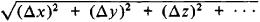Total Differential

Also found in: Dictionary, Wikipedia.
Related to Total Differential: Total differential equation

total differential

[′tōd·əl ‚dif·ə′ren·chəl]
(mathematics)
The total differential of a function of several variables, ƒ(x1, x2,…, xn ), is the function given by the sum of terms (∂ƒ/∂ xi ) dxi as i runs from 1 to n. Also known as differential.

Total Differential

The total differential of a function f(x, y, z, …) of several independent variables isDefine the increment of f as

∆ f = f(x +∆ x, y + ∆ y, z + ∆z,…)

- f(x, y, z,…)

then astends to zero, so does the difference Δf — df.

References in periodicals archive ?
This would indicate that EnergyPlus might be looking for total differential pressure as the input.
which have been obtained by taking the total differentials of equations in (1) and where A = [partial derivative]f/[partial derivative]x, B = [partial derivative]f/[partial derivative]u, C = [partial derivative]g/[partial derivative]x, and D = [partial derivative]g/[partial derivative]u.
17, an amount that represents approximately 14 percent of the total differential cost.
40, an amount that is identical to the total differential cost presented in Exhibit 1.
Therefore, the total differential phase shift for both field components is identical as long as the two bends are identical and this symmetry is preserved.
The total differential in the average log monthly earnings of the public and the informal sector is 0.
Over the time period of our analysis, we find a general decrease in the total differential between white males and all reference groups of around 30% (column 2 of Table 4).
A typical thermodynamic text usually incorporates calculus operators such as d, [delta] and [delta] to indicate total differential change, partial differential change or finite change respectively.
Pan-European motor insurance program as parent master contract, including fitness and total differential cover (DIC / DIL) for Arriva plc, Sunderland, a subsidiary of DB ML AG as well as for subsidiaries / majority-owned subsidiaries of Arriva plc in the countries named under Lot 1 and Lot 2 with underwriting and administrative control and management of the program in Germany.

Site: Follow: Share:
Open / Close